# Find electric potential and field from Electric Quadrupole

#### dreamspace

1. The problem statement, all variables and given/known data

So the problem revolves around a Linear Electric Quadrupole, with two positive charges on z-axis (in positions s and -s from origo) and two negative charges in origo.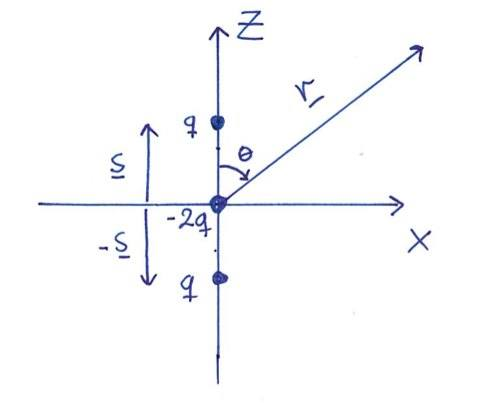1. Show that potential from a position R can be written as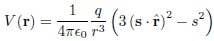2. The quadrupole moment can be written as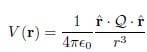where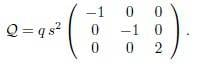Show that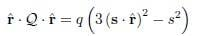Show that the electric field of the linear quadrupole can be written as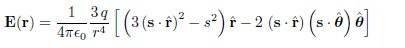We now change the Linear quadrupole out with a quadratic quadrupole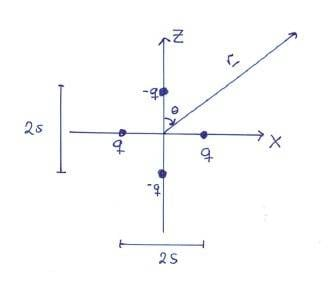Show that the quadrupole moment can be written aswhere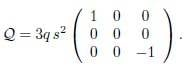2. Relevant equations

3. The attempt at a solution

I've done number 1. It was pretty straight forward, and I did it with Law of Cosines and some series expansion, but I'm completely stuck at number 2.

After some googling, it seems that there's tensors involved in this one, but I can't remember the professor talking about tensors for more than maybe 1 min, with a very brief example on Ohms Law (I think).

I just can't figure out where to start, as I have no intuitive image of how tensors work, or how they're to be applied in this problem.

As for the electric field, it should just be the negative Gradient of the potential.

But again, I can't get my head around tensors, or how to derive them from the picture or equations I've been given.

Last edited:

#### BvU

Homework Helper
Must have been quite some work, this number 1. Can you show it ?

In return I show how I work out $\hat r \cdot Q \cdot \hat r$ in cartesian coordinates: begin with Q.r (factor $q\,s^2$ I leave out and add at the end)
(I assume you are familiar with matrix multiplication) :
$$\left (\begin{array}{cc}-1&0&0\\0&-1&0\\0&0&2\end{array}\right )\, \left (\begin{array}{cc}\cos\phi \sin\theta\\ \sin\phi \sin\theta \\ \cos\theta\end{array}\right )=\left (\begin{array}{cc}-\cos\phi \sin\theta\\ -\sin\phi \sin\theta \\ 2\cos\theta\end{array}\right )$$ Then
$$\left (\begin{array}{cc}\cos\phi \sin\theta& \sin\phi \sin\theta & \cos\theta\end{array}\right ) \,\left (\begin{array}{cc}-\cos\phi \sin\theta\\ -\sin\phi \sin\theta \\ 2\cos\theta\end{array}\right ) = -\cos^2\phi \sin^2\theta - \sin^2\phi \sin^2\theta + 2 \cos^2\theta = - \sin^2\theta + 2 \cos^2\theta = 3 \cos^2\theta - 1$$ So that with $(s\cdot \hat r)^2= s^2 \cos^2\theta$ you get $$\hat r \cdot Q \cdot \hat r = q\,\left (3(s\cdot \hat r)^2 -s^2 \right )$$

And for now, no need for tensors of higher rank (tensors are arrays, matrices. Rank 0 is a scalar, rank 1 a vector, rank 2 a matrix -- I think I remember)

Last edited:

#### Taaben

I'm doing the exact same problem, and I've gotten to the quadratic quadrupole... Anyone know how to derive that stuff? Is it just rule of cosines for all of the charges, approximation with Taylor and plugging?

#### BvU

Homework Helper
Hello Taaben, and welcome to PF.
The derivation of this expansion can be googled, or found in books like Griffiths, Jackson, ...

It expands $1/|\vec r - \vec r' |$ in spherical harmonics. The nice thing is that for symmetries in the charge distributions (monopole, dipole, quadrupole) the series expansion of $\int \rho /|\vec r - \vec r' |$ terminates.

Sho how is our dreamspacer doing ?

### The Physics Forums Way

We Value Quality
• Topics based on mainstream science
• Proper English grammar and spelling
We Value Civility
• Positive and compassionate attitudes
• Patience while debating
We Value Productivity
• Disciplined to remain on-topic
• Recognition of own weaknesses
• Solo and co-op problem solving